• ## 单层LSTM和多层LSTM的输入与输出

千次阅读 多人点赞 2020-06-10 11:44:09
其实它是由一个LSTM单元的一个展开，如下图所示： 所以从左到右的每个LSTM Block只是对应一个时序中的不同的步。 在第一个图中，输入的时序特征有S个，长度记作：seq_len，每个特征是一个C维的向量,长度记作：input...
单层LSTM的输入与输出
RNN结构:对应的代码为：（代码中没写偏置）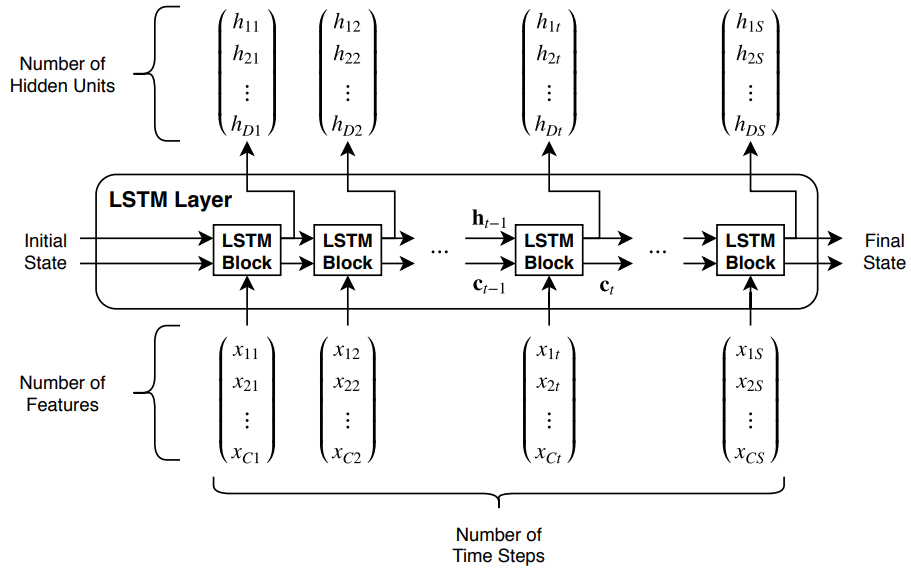上图是单层LSTM的输入输出结构图。其实它是由一个LSTM单元的一个展开，如下图所示：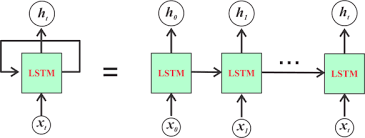所以从左到右的每个LSTM Block只是对应一个时序中的不同的步。
在第一个图中，输入的时序特征有S个，长度记作：seq_len，每个特征是一个C维的向量,长度记作：input_size。而Initial State是LSTM的隐藏状态和内部状态的一个输入的初始化。分别记作：h0和c0。
输出可以通过设置，来决定是输出所有时序步的输出，还是只输出最后一个时序步的输出。Final_State是隐藏状态和内部状态的输出，记作：hn和cn.
那么对于在pytorch中的函数LSTM的参数的输入情况如下：
输入数据：

X的格式：(seq_len,batch,input_size)
#batch是批次数，可以在LSTM()中设置batch_first，使得X的输入格式要求变为(batch，seq_len,input_size)
h0的格式：(1,batch,hidden_size)
c0的格式：(1,batch,hidden_size)
因为不管输入的数据X是多少个特征的，h0和c0的都只需要一个输入就行。

对于输出，输出的hidden_size的大小是由门控中的隐藏的神经元的个数来确定的。
输出的格式：

H的格式：(seq_len,batch,hidden_size)
#如果按照(seq_len,batch,hidden_size)  的格式输出，需要在LSTM()中设置return_sequences=True,否则默认只输出最后一个时间步的输出结果(1,batch,hidden_size).
hn的格式:(1,batch,hidden_size)
cn的格式:(1,batch,hidden_size)

这只是LSTM单元的输入输出格式，真实的其后还要跟一个全连接层，用于把LSTM的输出结果映射到自己想要的结果上，如分类：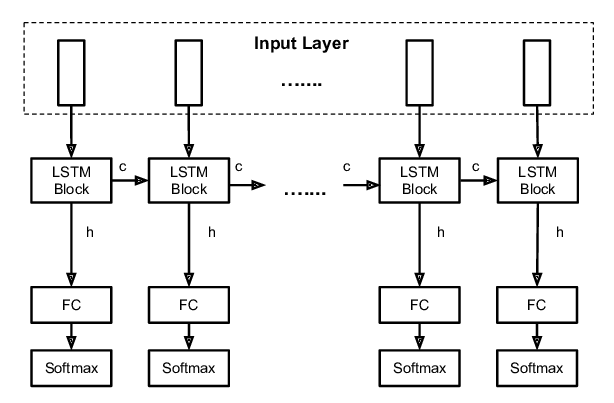如果只想要研究最后一个时间步的输出结果，只需在最后一个时间步添加全连接即可。
多层LSTM的输入与输出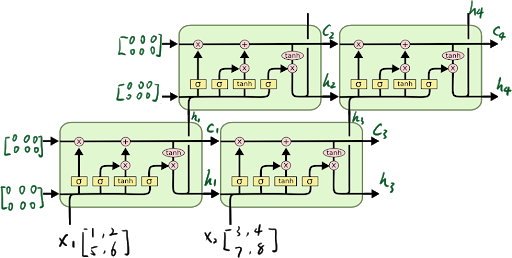对于多层的LSTM，需要把第一层的每个时间步的输出作为第二层的时间步的输入，如上图所示。
对于num_layers层LSTM：
输入数据：

X的格式：(seq_len,batch,input_size)
h0的格式：(num_layers,batch,hidden_size)
c0的格式：(num_layers,batch,hidden_size)

输出数据：

H的格式：(seq_len,batch,hidden_size)
hn的格式:(num_layers,batch,hidden_size)
cn的格式:(num_layers,batch,hidden_size)

如果是双向的，即在LSTM()函数中，添加关键字bidirectional=True，则：
单向则num_direction=1，双向则num_direction=2
输入数据格式：
input(seq_len, batch, input_size)
h0(num_layers * num_directions, batch, hidden_size)
c0(num_layers * num_directions, batch, hidden_size)
输出数据格式：
output(seq_len, batch, hidden_size * num_directions)
hn(num_layers * num_directions, batch, hidden_size)
cn(num_layers * num_directions, batch, hidden_size)
补充细节，下面是转载的：
版权声明：本文为CSDN博主「ssswill」的原创文章，遵循CC 4.0 BY-SA版权协议，转载请附上原文出处链接及本声明。
原文链接：https://blog.csdn.net/ssswill/article/details/88429794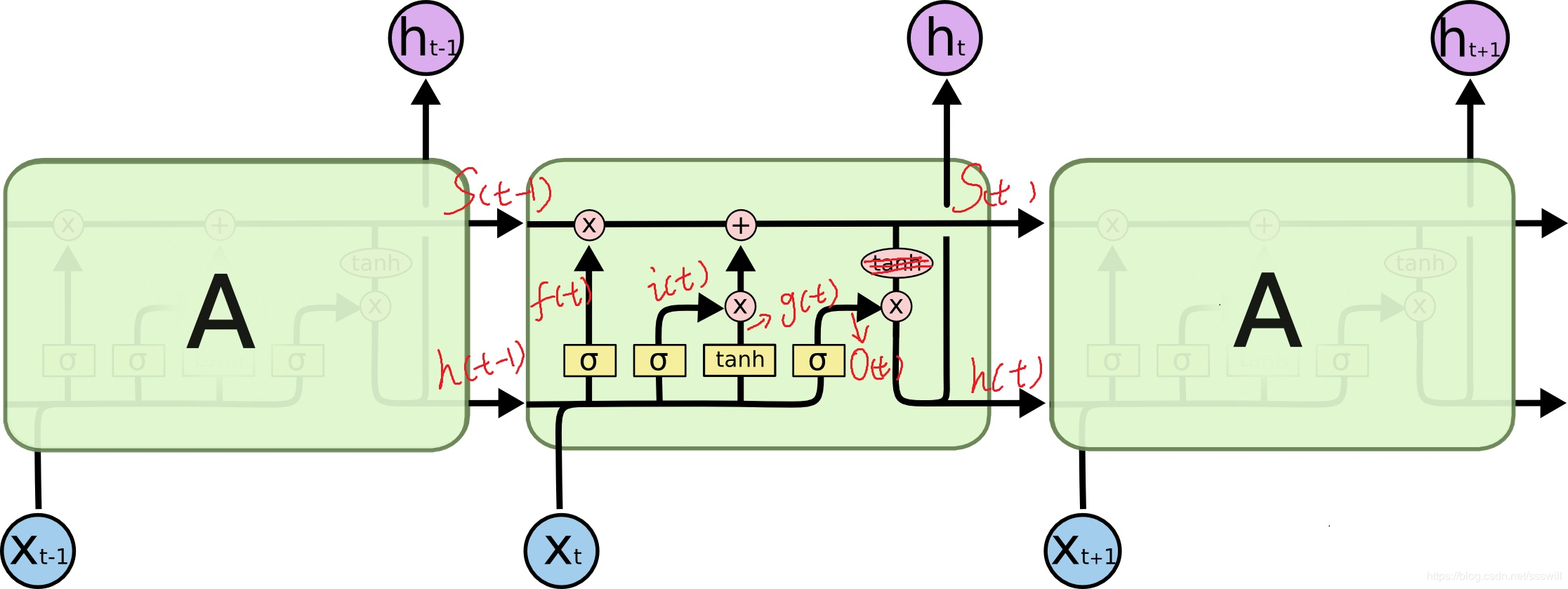输出的y=act(h_t*W_y)+b_y(图中未显示！)
可以看到中间的 cell 里面有四个黄色小框，你如果理解了那个代表的含义一切就明白了，每一个小黄框代表一个前馈网络层，对，就是经典的神经网络的结构，num_units就是这个层的隐藏神经元个数，就这么简单。其中1、2、4的激活函数是 sigmoid，第三个的激活函数是 tanh。
另外几个需要注意的地方：
1、 cell 的状态是一个向量，是有多个值的。
2、 上一次的状态 h(t-1)是怎么和下一次的输入 x(t) 结合（concat）起来的，这也是很多资料没有明白讲的地方，也很简单，concat， 直白的说就是把二者直接拼起来，比如 x是28位的向量，h(t-1)是128位的，那么拼起来就是156位的向量。
3、 cell 的权重是共享的，这是什么意思呢？这是指这张图片上有三个绿色的大框，代表三个 cell 对吧，但是实际上，它只是代表了一个 cell 在不同时序时候的状态，所有的数据只会通过一个 cell，然后不断更新它的权重。
4、那么一层的 LSTM 的参数有多少个？根据第 3 点的说明，我们知道参数的数量是由 cell 的数量决定的，这里只有一个 cell，所以参数的数量就是这个 cell 里面用到的参数个数。假设 num_units 是128，输入是28位的，那么根据上面的第 2 点，可以得到，四个小黄框的参数一共有 （128+28）*（128*4），也就是156 * 512，可以看看 TensorFlow 的最简单的 LSTM 的案例，中间层的参数就是这样，不过还要加上输出的时候的激活函数的参数，假设是10个类的话，就是128*10的 W 参数和10个bias 参数
5、cell 最上面的一条线的状态即 s(t) 代表了长时记忆，而下面的 h(t)则代表了工作记忆或短时记忆。
LSTM的训练过程：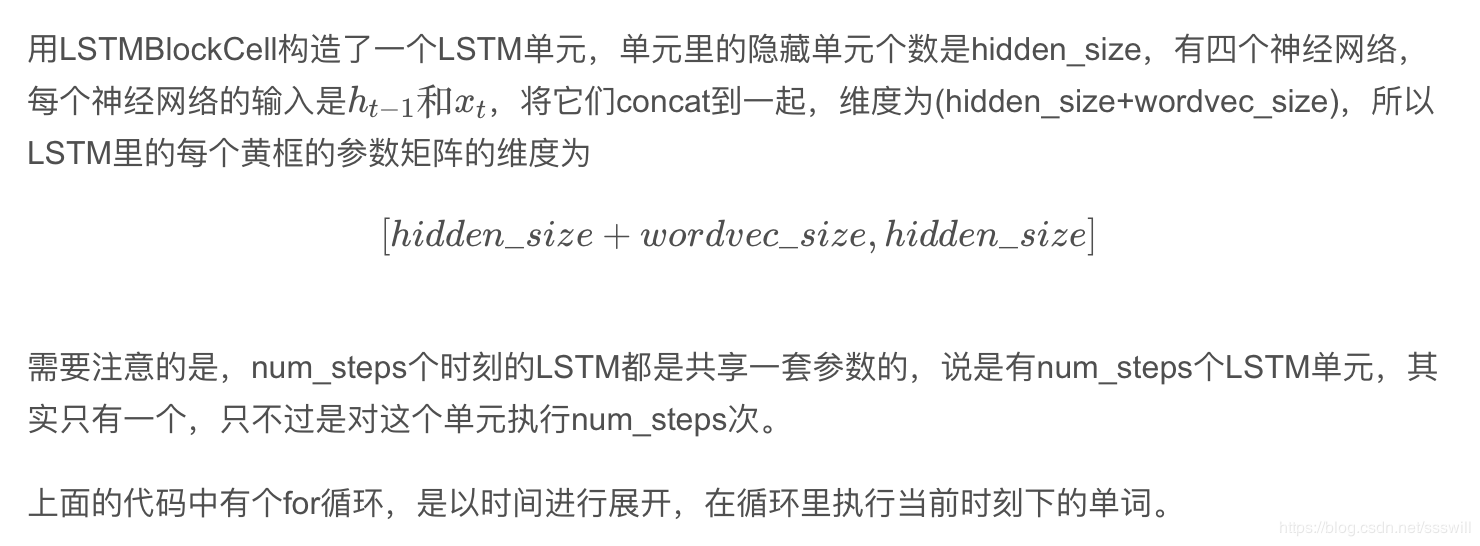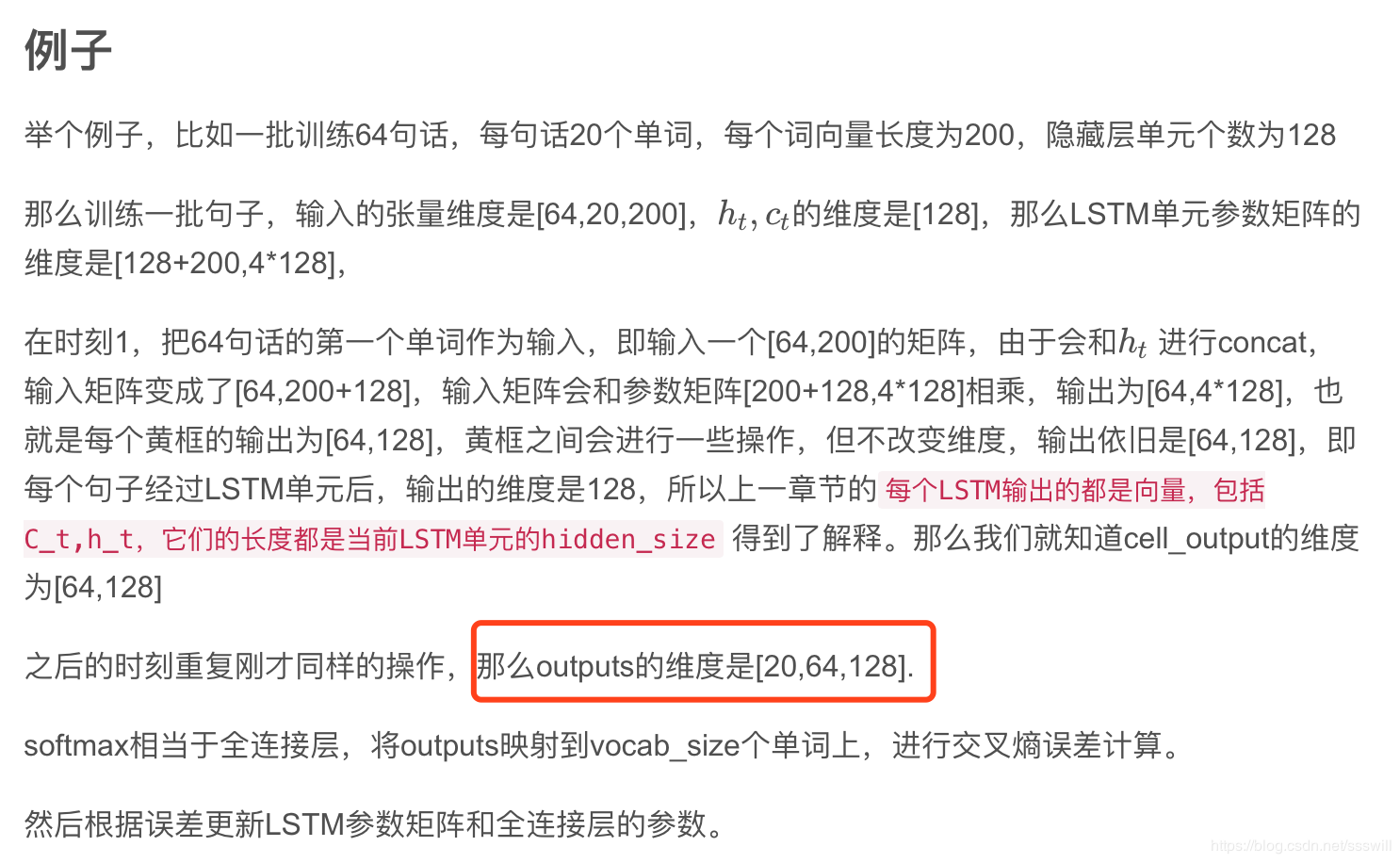说明：上面画红框的地方，如想输出如上的三维矩阵，需要指明参数：return_sequences=True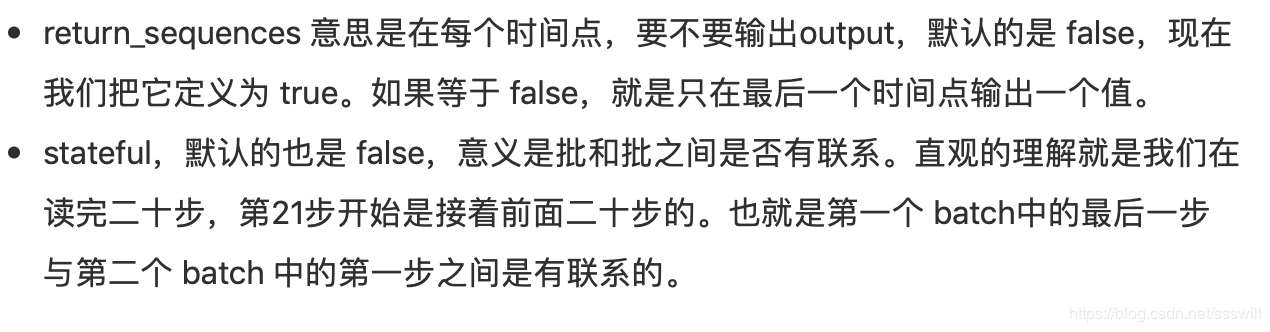再附一张图：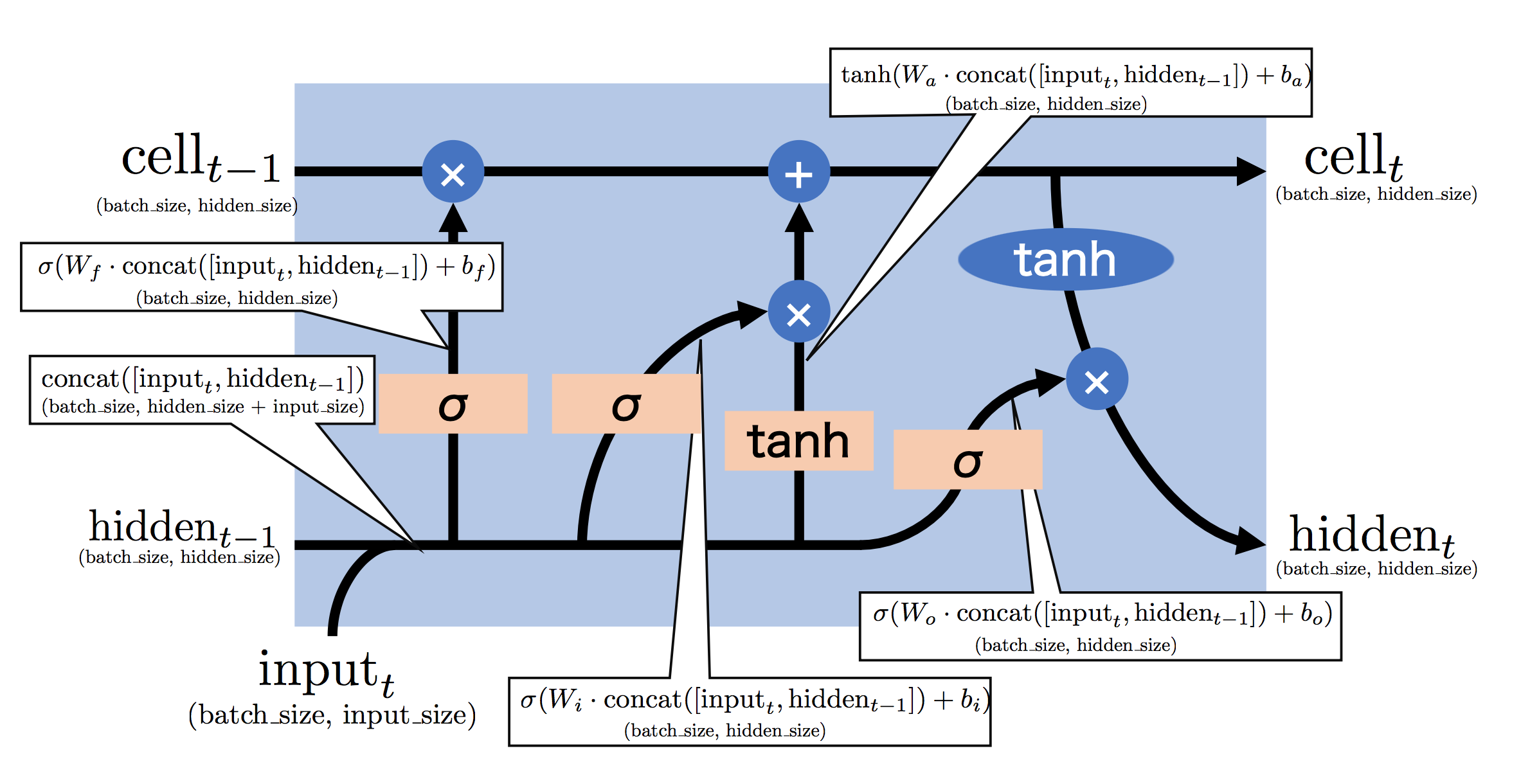白色框框中，第一行是实现细节，第二行是第一行输出结果的维度。
对于双向的Bi-LSTM网络：正向求得的第一个正h_1和反向求得的最后一个反h_-1,生成的结果进行对应位置相加，然后再经过act(W_y*h)+b_y得到对应的y.


展开全文rnn
• 多层LSTM需要每次都定义一新的BasicCell, 而不是定义一BasicCell之后次调用。 def lstm_model(X,Y,is_training): ###方式1：错误！！ #cell_unit = tf.nn.rnn_cell.BasicLSTMCell(HIDDEN_SIZ...


多层LSTM定义的两种方式，其中方式2是正确的，方式1会出现错误：

多层LSTM需要每次都定义一个新的BasicCell,    而不是定义一个BasicCell之后多次调用。

def lstm_model(X,Y,is_training):
###方式1：错误！！
#cell_unit = tf.nn.rnn_cell.BasicLSTMCell(HIDDEN_SIZE)
#cell = tf.nn.rnn_cell.MultiRNNCell([
#    cell_unit for _ in range(NUM_LAYERS)])
###方式2：正确
cell = tf.nn.rnn_cell.MultiRNNCell([
tf.nn.rnn_cell.BasicLSTMCell(HIDDEN_SIZE)
for _ in range(NUM_LAYERS)])

outputs, _ = tf.nn.dynamic_rnn(cell, X, dtype=tf.float32)

output = outputs[:,-1,:]

predictions = tf.contrib.layers.fully_connected(
output,1,activation_fn=None )
if not is_training:
return predictions,None,None

loss = tf.losses.mean_squared_error(labels=Y,predictions=predictions)

train_op = tf.contrib.layers.optimize_loss(
loss,tf.train.get_global_step(),
optimizer = 'Adagrad',learning_rate = 0.1)

return predictions,loss,train_op

完整示例程序的代码：

#-*-coding:utf-8-*-
import numpy as np
import tensorflow as tf

import matplotlib as mpl
mpl.use('Agg')
from matplotlib import pyplot as plt

HIDDEN_SIZE = 30
NUM_LAYERS = 2

TIME_STEPS = 10
TRAINING_STEPS = 10000
BATCH_SIZE = 32

TRAINING_EXAMPLES=10000
TESTING_EXAMPLES = 1000
SAMPLE_GAP = 0.01

def generate_data(seq):
X=[]#ninihaoniaho zh
Y=[]

for i in range(len(seq)-TIME_STEPS):
X.append([seq[i:i+TIME_STEPS]])
Y.append([seq[i+TIME_STEPS]])

return np.array(X,dtype=np.float32),np.array(Y,dtype=np.float32)

def lstm_model(X,Y,is_training):
# cell_unit = tf.nn.rnn_cell.BasicLSTMCell(HIDDEN_SIZE)
cell = tf.nn.rnn_cell.MultiRNNCell([
tf.nn.rnn_cell.BasicLSTMCell(HIDDEN_SIZE)
for _ in range(NUM_LAYERS)])
outputs, _ = tf.nn.dynamic_rnn(cell, X, dtype=tf.float32)

output = outputs[:,-1,:]

predictions = tf.contrib.layers.fully_connected(
output,1,activation_fn=None )
if not is_training:
return predictions,None,None

loss = tf.losses.mean_squared_error(labels=Y,predictions=predictions)

train_op = tf.contrib.layers.optimize_loss(
loss,tf.train.get_global_step(),
optimizer = 'Adagrad',learning_rate = 0.1)

return predictions,loss,train_op

def train(sess,train_X,train_Y):
ds=tf.data.Dataset.from_tensor_slices((train_X,train_Y))
ds = ds.repeat().shuffle(1000).batch(BATCH_SIZE)
X,Y = ds.make_one_shot_iterator().get_next()

### use model get result
with tf.variable_scope('model'):
predictions,loss,train_op = lstm_model(X,Y,True)

sess.run(tf.global_variables_initializer())
for i in range(TRAINING_STEPS):
_,l = sess.run([train_op,loss])
if i%100 ==0:
print('train step:'+str(i) + ',loss:'+str(l))

def run_eval(sess, test_X, test_y):
# print('------------runned')
# 将测试数据以数据集的方式提供给计算图。
ds = tf.data.Dataset.from_tensor_slices((test_X, test_y))
ds = ds.batch(1)
X, y = ds.make_one_shot_iterator().get_next()

# 调用模型得到计算结果。这里不需要输入真实的y值。
with tf.variable_scope("model", reuse=True):
prediction, _, _ = lstm_model(X, [0.0], False)

# 将预测结果存入一个数组。
predictions = []
labels = []
for i in range(TESTING_EXAMPLES):
p, l = sess.run([prediction, y])
predictions.append(p)
labels.append(l)

# 计算rmse作为评价指标。
predictions = np.array(predictions).squeeze()
labels = np.array(labels).squeeze()
rmse = np.sqrt(((predictions - labels) ** 2).mean(axis=0))
print("Root Mean Square Error is: %f" % rmse)

# 对预测的sin函数曲线进行绘图。
plt.figure()
plt.plot(predictions, label='predictions')
plt.plot(labels, label='real_sin')
plt.legend()
plt.show()

test_start = (TRAINING_EXAMPLES+TIME_STEPS)*SAMPLE_GAP
test_end = test_start + (TESTING_EXAMPLES+TIME_STEPS)*SAMPLE_GAP

train_X,train_Y = generate_data(np.sin(np.linspace(
test_start,test_end,TRAINING_EXAMPLES+TIME_STEPS,dtype=np.float32)))
test_X,test_Y = generate_data(np.sin(np.linspace(
test_start,test_end,TESTING_EXAMPLES+TIME_STEPS,dtype=np.float32)))

with tf.Session() as sess:
train(sess,train_X,train_Y)
run_eval(sess,test_X,test_Y)




展开全文• 堆叠LSTM（Stacked LSTM）是此模型的扩展，具有多个LSTM层。 文章结构 为什么要堆叠LSTM？ 堆叠LSTM结构 堆叠LSTM的Tensorflow实现 为什么要堆叠LSTM？ 堆叠LSTM使模型深度更深，提取的特征更深层次，从而使预测...
引言
原始LSTM模型由单个LSTM层和随后的输出层组成。 堆叠LSTM（Stacked LSTM）是此模型的扩展，具有多个LSTM层。关于LSTM相关知识请看这片文章：LSTM（长短时记忆网络）详解
文章结构

为什么要堆叠LSTM？
堆叠LSTM结构
堆叠LSTM的Tensorflow实现

为什么要堆叠LSTM？
堆叠LSTM使模型深度更深，提取的特征更深层次，从而使预测更准确。堆叠LSTM在大范围的预测问题上取得了不错的效果。

The success of deep neural networks is commonly attributed to the hierarchy that is introduced due to the several layers. Each layer processes some part of the task we wish to solve, and passes it on to the next. In this sense, the DNN can be seen as a processing pipeline, in which each layer solves a part of the task before passing it on to the next, until finally the last layer provides the output.— Training and Analyzing Deep Recurrent Neural Networks, 2013

Deep learning is built around a hypothesis that a deep, hierarchical model can be exponentially more efficient at representing some functions than a shallow one.— How to Construct Deep Recurrent Neural Networks, 2013.

堆叠LSTM的结构图1 两层LSTM逻辑结构图2 三层LSTM时序结构

堆叠LSTM其实就是把前一层LSTM的输出当作后一层LSTM的输入，送入网络中。

堆叠LSTM的Tensorflow实现
#2021年5月20日14:14:15
#LSTM预测手写数字MNIST数据集
#%tensorflow_version 1.x
import tensorflow as tf

from tensorflow.examples.tutorials.mnist import input_data
#tf.reset_default_graph()
"""加载数据"""
mnist = input_data.read_data_sets('MNIST_data', one_hot=True)

"""参数设置"""
BATCH_SIZE = 128        # BATCH的大小，相当于一次处理128个image
TIME_STEP = 28         # 一个LSTM中，输入序列的长度，image有28行
INPUT_SIZE = 28         # x_i 的向量长度，image有28列
LR = 0.001           # 学习率
NUM_UNITS = 128         # LSTM的输出维度
ITERATIONS = 10000         # 迭代次数
N_CLASSES = 10            # 输出大小，0-9十个数字的概率
"""定义计算"""
# 定义 placeholders 以便接收x,y
# 维度是[BATCH_SIZE，TIME_STEP * INPUT_SIZE]
train_x = tf.placeholder(tf.float32, [BATCH_SIZE, TIME_STEP * INPUT_SIZE])

# 输入的是二维数据，将其还原为三维，维度是[BATCH_SIZE, TIME_STEP, INPUT_SIZE]
image = tf.reshape(train_x, [BATCH_SIZE, TIME_STEP, INPUT_SIZE])
train_y = tf.placeholder(tf.int32, [BATCH_SIZE, N_CLASSES])

# 定义网络结构两层lSTM
rnn_cell = tf.contrib.rnn.BasicLSTMCell(num_units=NUM_UNITS*2)
rnn_cell_ = tf.contrib.rnn.BasicLSTMCell(num_units=NUM_UNITS)
multi_cell = tf.contrib.rnn.MultiRNNCell([rnn_cell, rnn_cell_],state_is_tuple=True)
outputs, final_state = tf.nn.dynamic_rnn(
cell=multi_cell,              # 选择传入的cell
inputs=image,               # 传入的数据
initial_state=None,         # 初始状态
dtype=tf.float32,           # 数据类型
# False: (batch, time_step, x_input); True: (time_step,batch,x_input)，
# 这里根据image结构选择False
# If false, these Tensors must be shaped [batch_size, max_time, depth].
time_major=False,
)

"""获取输出"""
output = tf.layers.dense(
inputs=outputs[:, -1, :], units=N_CLASSES)  # 取最后一路输出送入全连接层

"""定义损失和优化方法"""
loss = tf.losses.softmax_cross_entropy(
onehot_labels=train_y,
logits=output)      # 计算loss

train_op = tf.train.AdamOptimizer(LR).minimize(loss)  # 选择优化方法

correct_prediction = tf.equal(
tf.argmax(
train_y, axis=1), tf.argmax(
output, axis=1))

accuracy = tf.reduce_mean(tf.cast(correct_prediction, 'float'))  # 计算正确率
saver = tf.train.Saver() #保存模型
"""summary"""
tf.summary.scalar('loss_train',loss)
tf.summary.scalar('loss_val',loss)
tf.summary.scalar('acc',accuracy)
merge_summary_train = tf.summary.merge([tf.get_collection(tf.GraphKeys.SUMMARIES,'loss_train')])
merge_summary_val = tf.summary.merge([tf.get_collection(tf.GraphKeys.SUMMARIES,'loss_val'),
tf.get_collection(tf.GraphKeys.SUMMARIES,'acc')])

"""训练"""
with tf.Session() as sess:
sess.run(tf.global_variables_initializer())     # 初始化计算图中的变量
train_writer = tf.summary.FileWriter('/content/drive/MyDrive/LSTM_MNIST_multi/train',sess.graph)
val_writer = tf.summary.FileWriter('/content/drive/MyDrive/LSTM_MNIST_multi/val',sess.graph)
for step in range(1, ITERATIONS):    # 开始训练
x, y = mnist.train.next_batch(BATCH_SIZE)
_, loss_ = sess.run([train_op, loss], {train_x: x, train_y: y})
train_summary = sess.run(merge_summary_train,
feed_dict={train_x: x, train_y: y})
train_writer.add_summary(train_summary,step)
if step % 100 == 0:      # test（validation）
saver.save(sess, "Model/LSTM_MNIST_" + str(step))
test_x, test_y = mnist.test.next_batch(BATCH_SIZE)
accuracy_ = sess.run(accuracy, {train_x: test_x, train_y: test_y})
val_summary = sess.run(merge_summary_val,
{train_x: x, train_y: y})
val_writer.add_summary(val_summary,step)
print(
'train loss: %f' %
loss_,
'| validation accuracy: %f' %
accuracy_)
train_writer.close()
val_writer.close()


参考：
Stacked Long Short-Term Memory Networks
Training and Analyzing Deep Recurrent Neural Networks——Michiel Hermans, Benjamin Schrauwen


展开全文tensorflow 深度学习 神经网络 人工智能
• LSTM增加了一种携带信息跨越多个时间步的方法，假设有一条平行于你所处理的序列的传送带，能在任意时刻把重要的信息跳上传送带传到需要的时候再跳回来，它保存信息以便后面使用，从而防止较早的信息在处理过程中逐渐...
RNN无法记住很多个时间步长之前出现过的信息，即长期依赖问题，也无法提取之前有效的记忆和存入当前有效的记忆，因此我们在记忆体内增加了一个记忆细胞来避免长期依赖问题。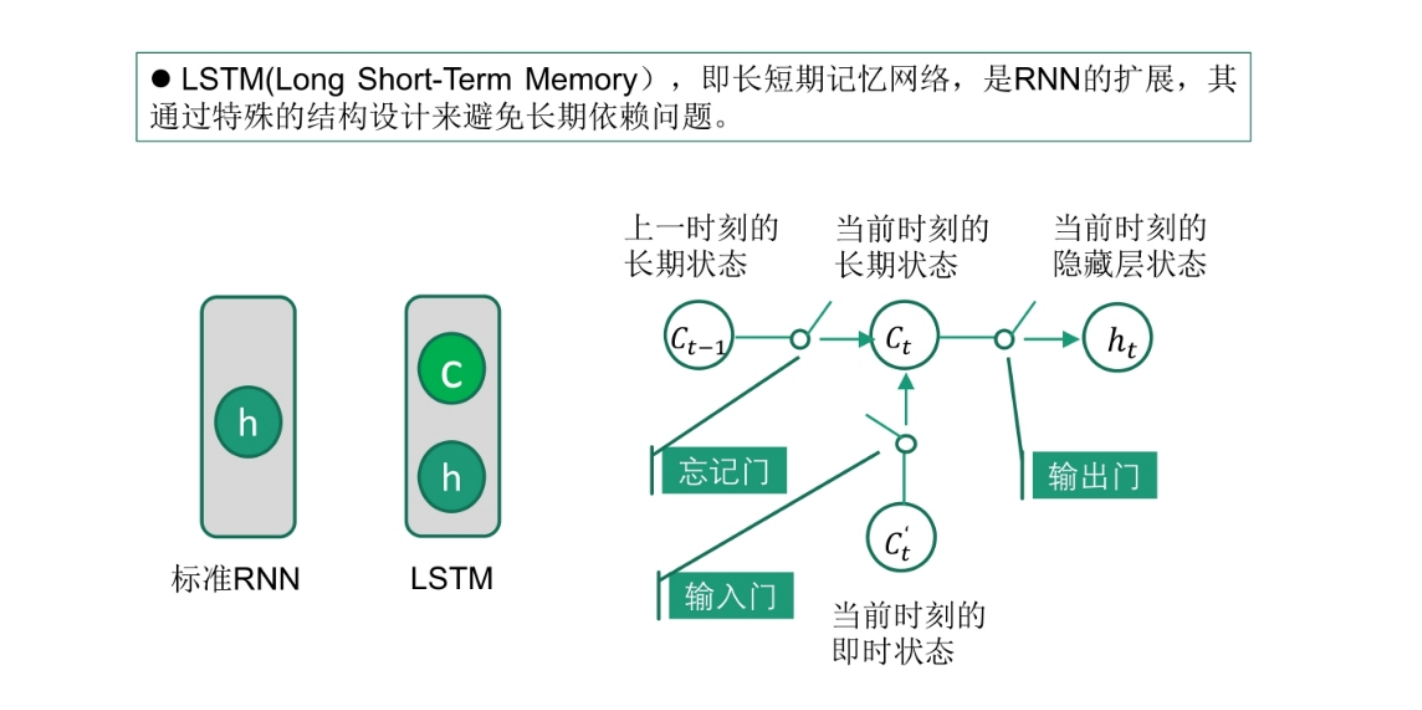LSTM增加了一种携带信息跨越多个时间步的方法，假设有一条平行于你所处理的序列的传送带，能在任意时刻把重要的信息跳上传送带传到需要的时候再跳回来，它保存信息以便后面使用，从而防止较早的信息在处理过程中逐渐消失
LSTM的整体框架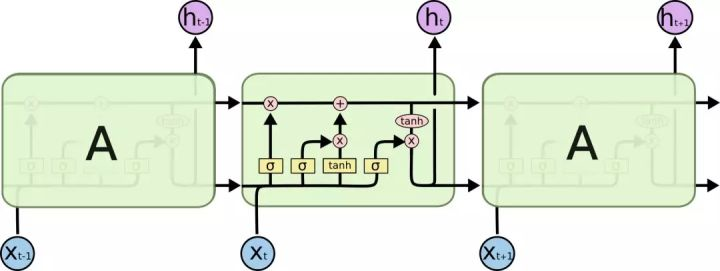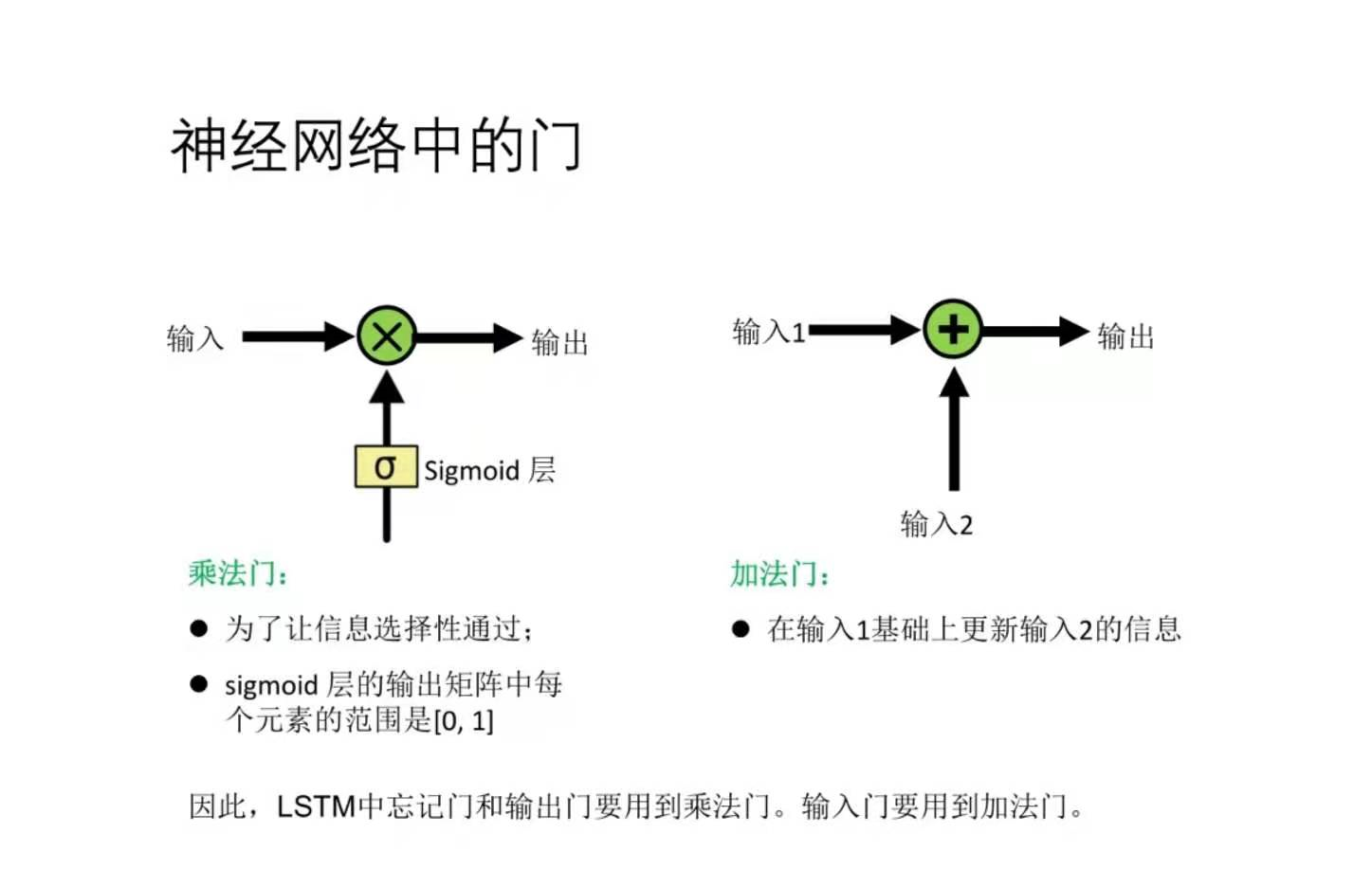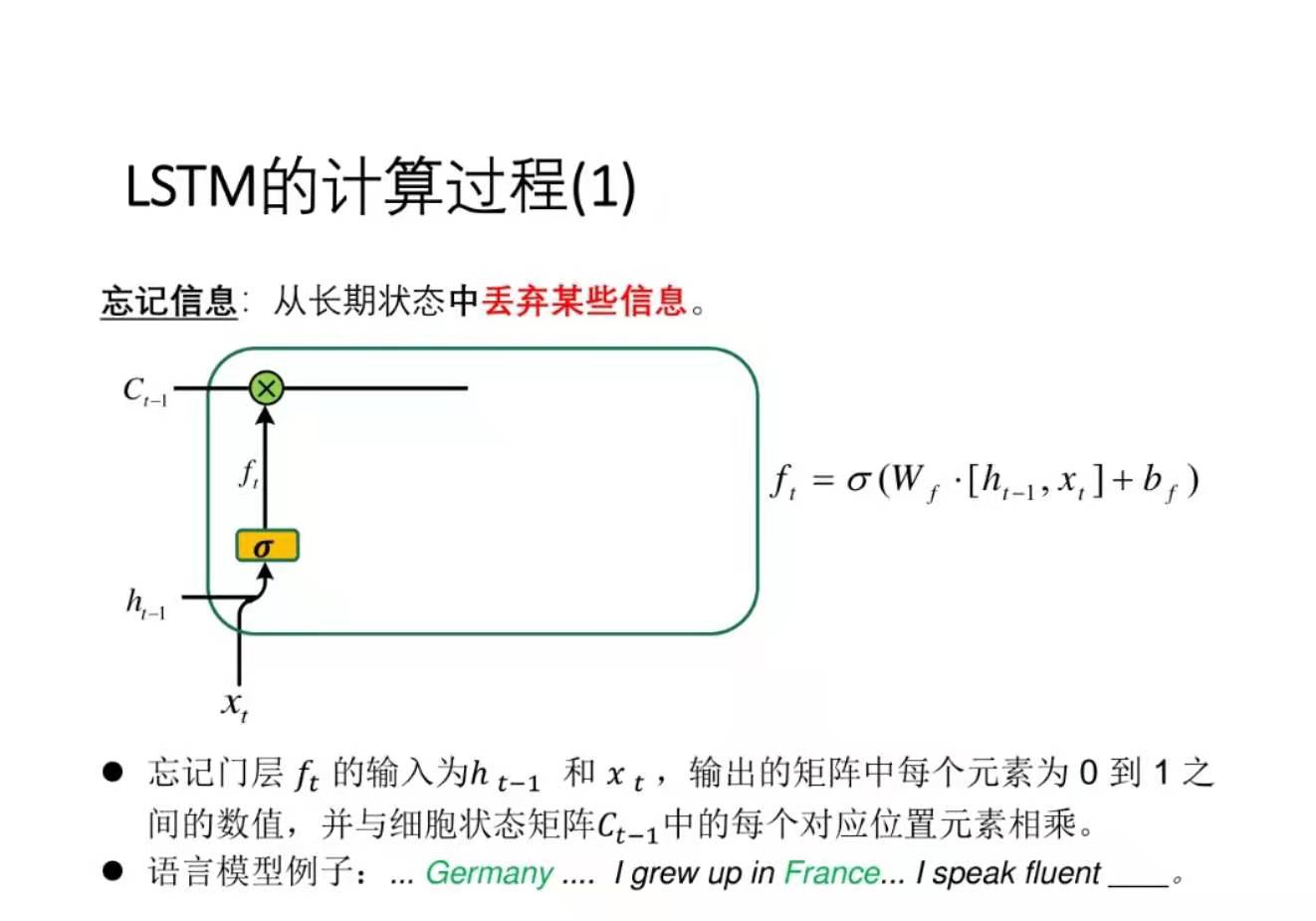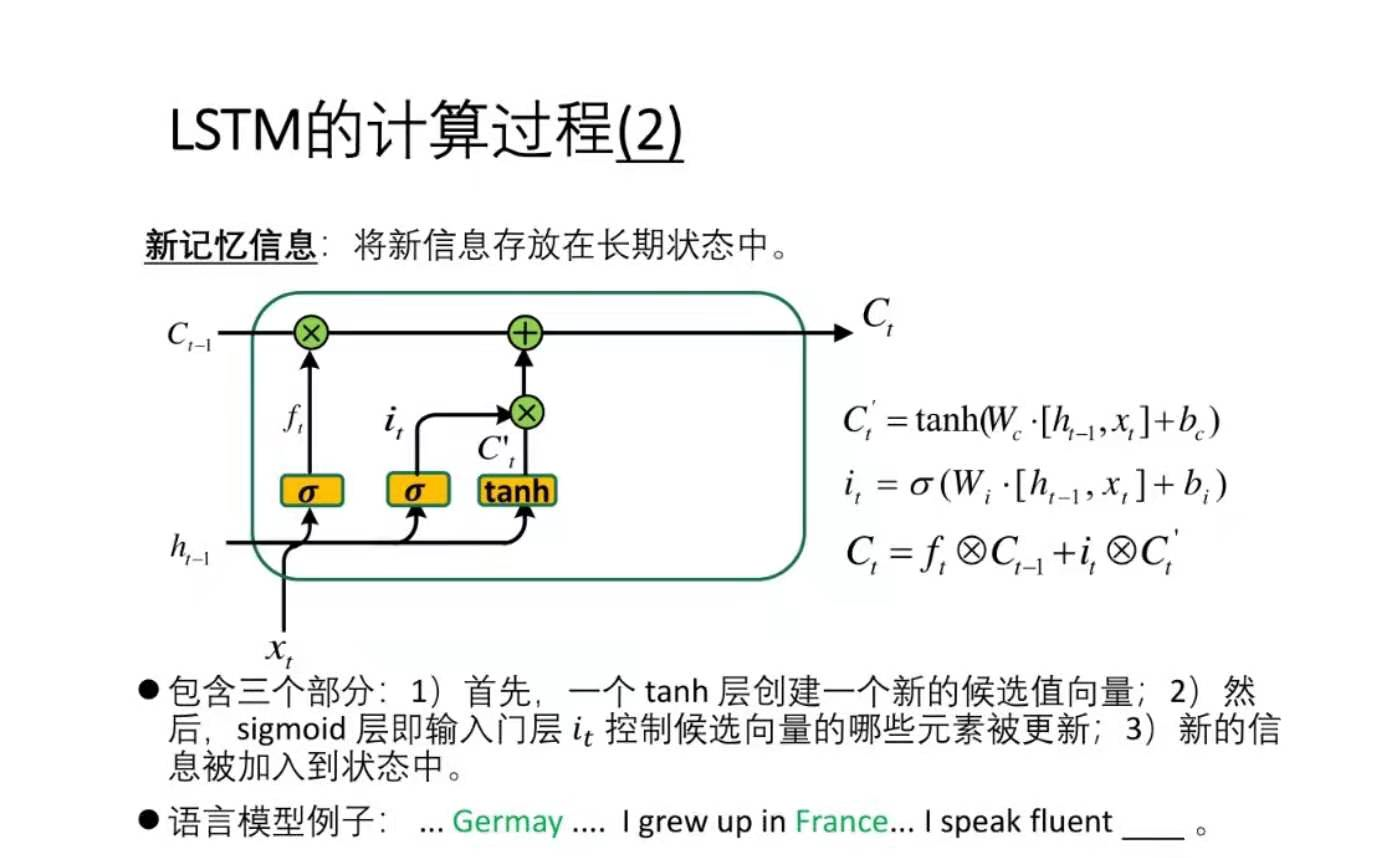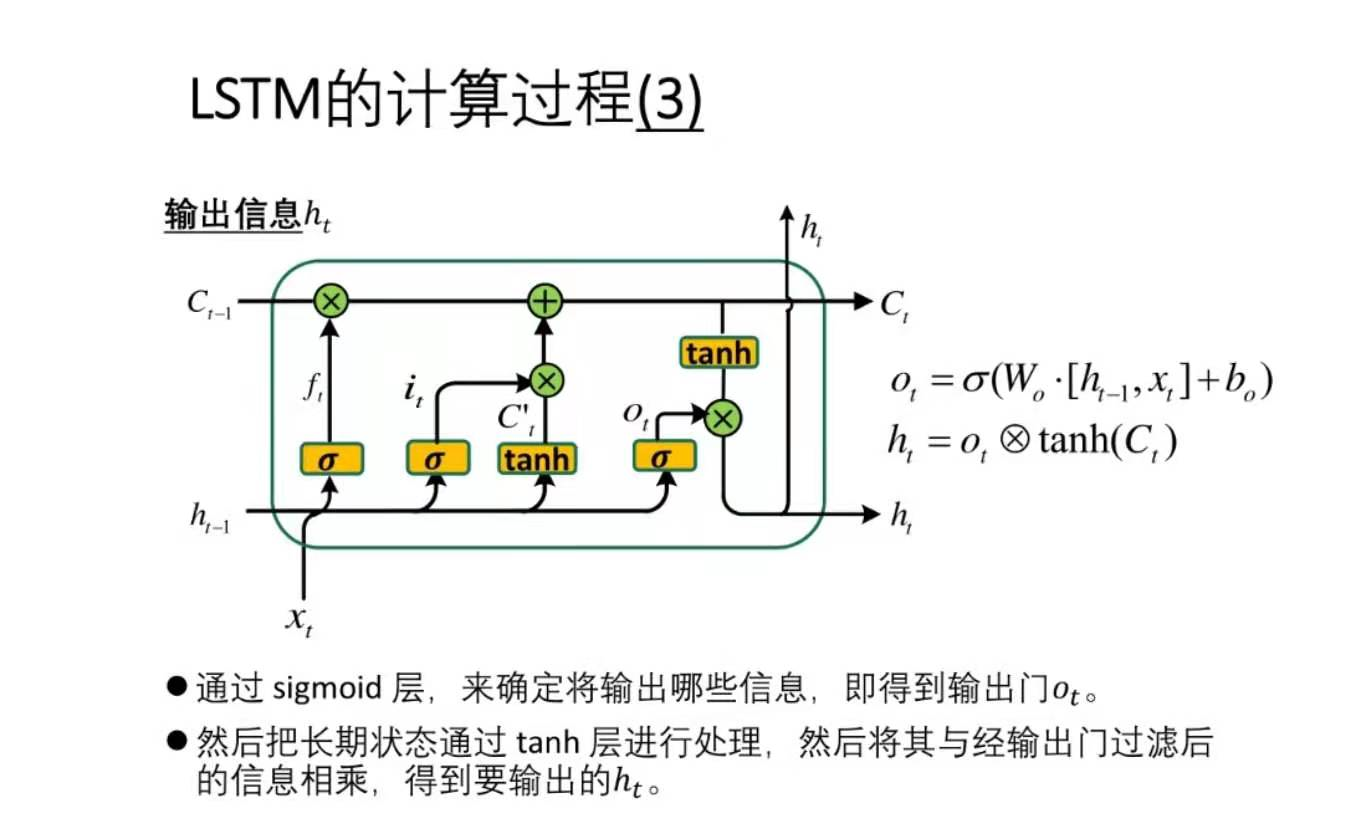用σ(sigmoid)函数控制信息保留程度，1为全保留，0为全抛弃
用tanh函数把数据处理成候选值向量
记忆门，输入门，输出门的循环权重和偏置的值不同
用LSTM预测实现股票预测
import tensorflow as tf
import numpy as np
from tensorflow.keras.layers import  Dropout,Dense,LSTM
import matplotlib.pyplot as plt
import os
import  pandas as pd
from sklearn.preprocessing import  MinMaxScaler
from sklearn.metrics import  mean_squared_error,mean_absolute_error
import math
maotai=pd.read_csv('./SH600519')#读取股票文件
training_set=maotai.iloc[0:2426-300, 2:3].values #前[2426-300=2126]天的开盘价作为训练集，表格从0开始计数，2:3是提取[2:3]列，前必后开
test_set=maotai.iloc[2426-300:,2:3].values#后300天的开盘价作为测试集
#归一化
sc=MinMaxScaler(feature_range=(0,1))#定义归一化：归一化到（0,1）之间
training_set_scaled=sc.fit_transform(training_set)#求得训练集的最大值，最小值这些训练集固有的属性，并在训练集上进行归一化
test_set=sc.transform(test_set)#利用训练集的属性对测试集进行归一化
x_train=[]
y_train=[]
x_test=[]
y_test=[]
#利用for循环，遍历整个训练集，提取训练集中连续60天的开盘价作为输入特征x_train，第61天的数据作为标签，for循环共构建2426-300-60=2066组数据
for i in range(60,len(training_set_scaled)):
x_train.append(training_set_scaled[i-60:i,0])
y_train.append(training_set_scaled[i,0])
#打乱训练顺序
np.random.seed(7)
np.random.shuffle(x_train)
np.random.seed(7)
np.random.shuffle(y_train)
tf.random.set_seed(7)
#将训练集由list格式转换成array格式
x_train,y_train=np.array(x_train),np.array(y_train)
#使输入的训练特征符合要求
x_train=np.reshape(x_train,(x_train.shape,60,1))
#测试集前60天为x_train,第61天为标签，遍历后300天数据
for i in range(60,len(test_set)):
x_test.append(test_set[i-60:i,0])
y_test.append(test_set[i,0])
x_test,y_test=np.array(x_test),np.array(y_test)
x_test=np.reshape(x_test,(x_test.shape,60,1))
model=tf.keras.Sequential([
LSTM(80,return_sequences=True),Dropout(0.2),
LSTM(100),
Dropout(0.2),
Dense(1)
])
model.compile(optimizer=tf.keras.optimizers.Adam(0.001),
loss='mean_squared_error')#损失函数为均方误差
checkpoint_save_path="./checkpoint/stock.ckpt"
if os.path.exists(checkpoint_save_path+'.index'):
print('----------load the model--------------')
model.load_weights(checkpoint_save_path)
cp_callback=tf.keras.callbacks.ModelCheckpoint(filepath=checkpoint_save_path,save_weights_only=True,
save_best_only=True,
monitor='val_loss')
history=model.fit(x_train,y_train,batch_size=64,epochs=50,validation_data=(x_test,y_test),
validation_freq=1,callbacks=[cp_callback])#执行训练过程
model.summary()
file=open('./weights.txt','w')#参数提取
for v in model.trainable_variables:
file.write(str(v.name)+'\n')
file.write(str(v.shape)+'\n')
file.write(str(v.numpy())+'\n')
file.close()
loss=history.history['loss']
val_loss=history.history['val_loss']
plt.plot(loss,label='Training Loss')
plt.plot(val_loss,label='Validation Loss')
plt.title('Training and Validation Loss')
plt.legend()
plt.show()
#测试集输入模型进行预测
predicted_stock_price=model.predict(x_test)
#对预测数据进行还原---从（0,1）反归一化到原始范围
predicted_stock_price=sc.inverse_transform(predicted_stock_price)
#对真实数据还原
real_stock_price=sc.inverse_transform(test_set[60:])
#画出真实数据和预测数据的对比曲线
plt.plot(real_stock_price,color='red',label='MaoTai Stock Price')
plt.plot(predicted_stock_price,color='blue',label='Predicted Maotai Stock Price')
plt.title('MaoTai Stock Price Prediction')
plt.xlabel('Time')
plt.ylabel('MaoTai Stock Price')
plt.legend()
plt.show()
mse=mean_squared_error(predicted_stock_price,real_stock_price)#求均方误差
rmse=math.sqrt(mean_squared_error(predicted_stock_price,real_stock_price))#标准差
mae=mean_absolute_error(predicted_stock_price,real_stock_price)#绝对误差
print('均方误差：%.6f'%mse)
print('均方根误差：%.6f'%rmse)
print('平均绝对误差：%.6f'%mae)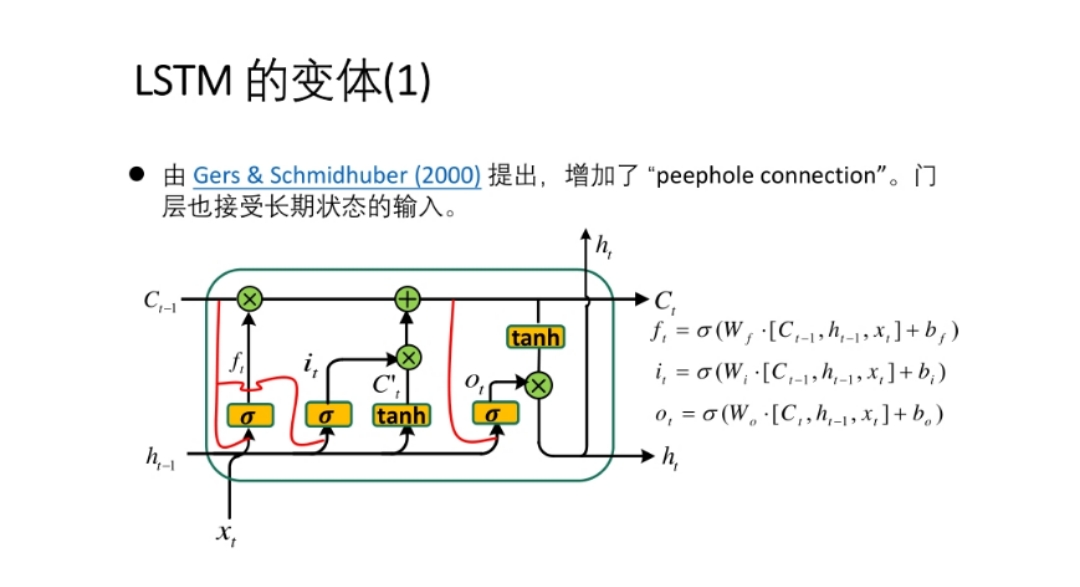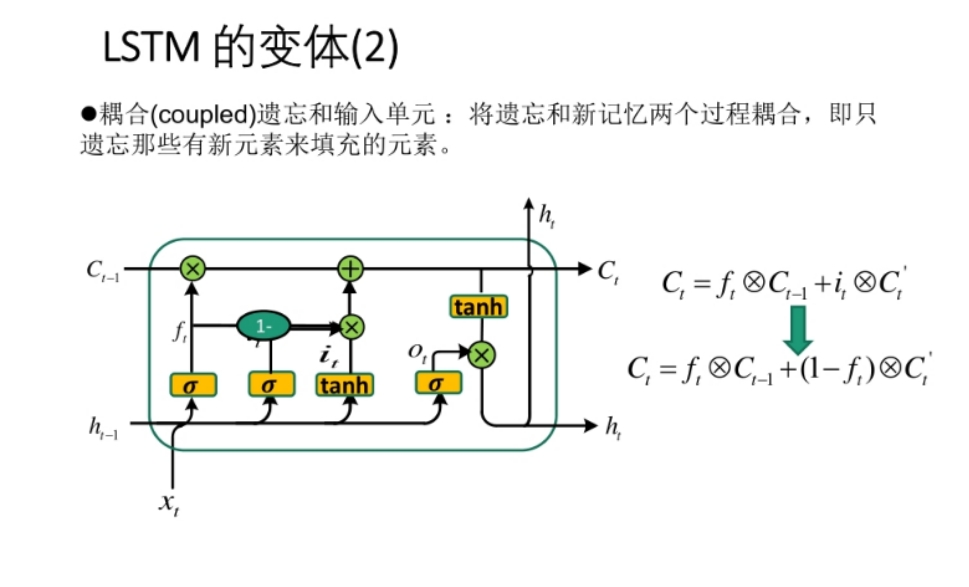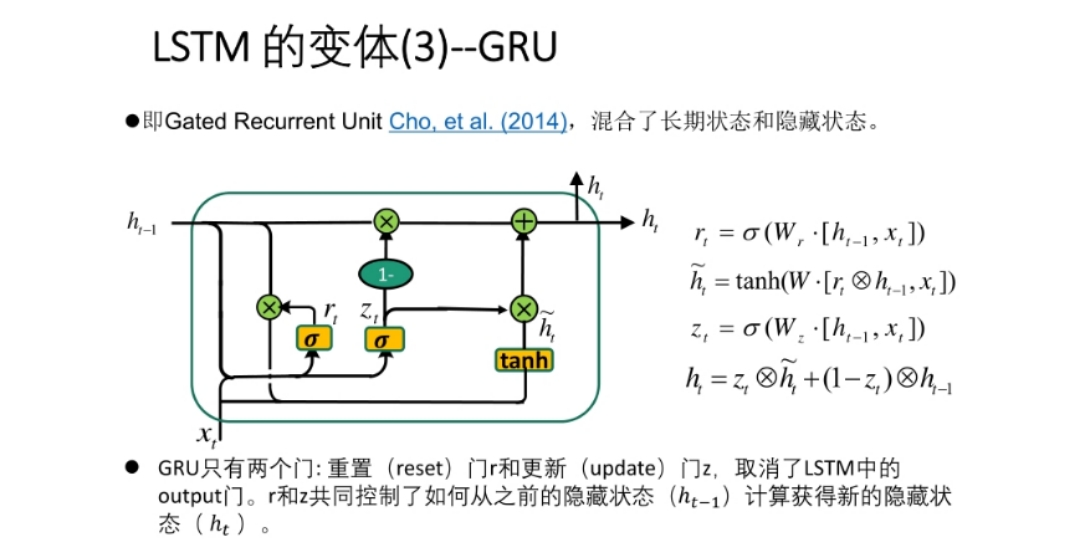展开全文自然语言处理 深度学习 tensorflow
• 有些时候，主要考虑的是哪些输入，有时候，考虑的是（输入，输出）之间的模型； 一、RNN f,g 就是普通的激活函数，可能是tanh；...多层RNN中，第一的输入是Ht1~ (Xt, Ht-1)，后面的输入不再...
• ## 多层 LSTM的实现

千次阅读 2019-02-13 20:10:51
前言: 根据我本人学习 TensorFlow 实现 LSTM 的经历，发现网上虽然也有不少教程，其中很都是根据官方给出的例子，用多层 LSTM 来实现 PTBModel 语言模型，比如： tensorflow笔记：多层LSTM代码分析 但是感觉...
• model = Sequential() model.add(LSTM(32, batch_input_...在很LSTM的框图中，我们都会将LSTM单元按照时间顺序排列开来，句子的长度是多少我们就会画出多少个LSTM单元。这也是给我们带来误解的地方。实际上一LST...神经网络
• ## 多层LSTM结构的深入解读

万次阅读 多人点赞 2018-06-15 01:37:52
读这篇文章的时候，默认你已经对LSTM神经网络有了一初步的认识，当你深入理解时，可能会对多层LSTM内部的隐藏节点数，有关cell的定义或者每一的输入输出是什么样子的特别好奇，虽然神经网络就像是一黑箱子一样...
• 特别的是，输入到下一个LSTM层的输入，是那一个特定层的前一个状态， 隐藏的前一层的激活层也是 [这尼玛说的是啥？]我们要 把每一层的states保存起来，将会有很多个LSTMTuples， 为了方便，我们会用一个整的状态...
• 输入格式：batch_size*784改成batch_size*28*28,28序列，内容是一行的28灰度数值。 让神经网络逐行扫描一手写字体图案，总结各行特征，通过时间序列串联起来，最终得出结论。 网络定义：单独定义一获取...tensorflow state
• Matlab多层lstm代码使用具有CNN功能的深度双向LSTM在视频序列中进行动作识别 我们已经使用caffe模式使用matlab脚本“ oneFileFeatures ...”从视频中提取了深层功能。 每CSV文件代表一视频的功能。 使用“ ...
• https://blog.csdn.net/Jerr__y/article/details/61195257?depth_1-utm_source=distribute.pc_relevant.none-task-blog-BlogCommendFromMachineLearnPai2-1&utm_source=distribute.pc_relevant.none-task-blog-B...
• 第二是实现两个LSTM的state的concat操作, 分析 state 的结构. 对于第一个问题,之前一直没有注意过, 看下面两个例子: 在这里插入代码片 import tensorflow as tf num_units = [20, 20] #Unit1, OK # X = tf.random_...
• 多层双向LSTM with Attention对多层双向LSTM模型做了一些改进，不再单纯地只利用最后时刻的隐藏状态（两方向最后时刻隐藏状态拼接）进行分类，而是考虑每时间步的隐藏状态，对每时间步的隐藏状...文本分类
• 对于输入文本序列，在LSTM的每时间步输入序列中一单词的嵌入表示，计算当前时间步的隐藏状态，用于当前时间步的输出以及传递给下一时间步和下一 单词的词向量一起作为LSTM单元输入，然后再计算下一时间步...文本分类
• 1.tf.Graph() ...添加一操作到默认的图里面，只要简单的调用一定义了新操作的函数就行。比如下面的例子展示的： import tensorflow as tf import numpy as np c=tf.constant(value=1...
• 常用的LSTM，或是双向LSTM，输出的结果通常是以下两： 1） outputs，包括所有节点的hidden 2） 末节点的state，包括末节点的hidden和cell 大部分任务有这些就足够了，state是随着节点间信息的传递依次变化并...tensorflow states
• 前言: 根据我本人学习 TensorFlow 实现 LSTM 的经历，发现网上虽然也有不少教程，其中很都是根据官方给出的例子，用多层 LSTM 来实现 PTBModel 语言模型，比如： tensorflow笔记：多层LSTM代码分析 但是感觉...机器学习 深度学习 tensorflow 入门
• 堆叠式LSTM架构可以定义为由多个LSTM层组成的LSTM模型。上面的LSTM层提供序列输出而不是单个值输出到下面的LSTM层。具体地说，每个输入时间步长一个输出，而不是所有输入时间步长的一个输出时间步长。 ...
• import tensorflow as tf import numpy as np import matplotlib as mpl from matplotlib import ...hidden_size = 30 # LSTM 中隐藏节点的个数 //TODO 这size是是什么意思 num_layers = 2 # LSTM数 timest...tensorflow 序列分析
• 从pytorch代码角度初次理解LSTM各种术语。 LSTM: ...hidden_size 隐状态的维数：（每个LSTM单元或者时间步的输出的ht的维度，单元内部有权重与偏差计算） num_layers RNN的个数：（在竖直...RNN
• 在TensorFlow中用BasicLSTMCell和MultiRNNCell实现多层LSTM循环神经网络时，关于MultiRNNCell的用法网络上很例程中是错误的，误人子弟 下面这样直接用一BasicLSTMCell复制是错误的，会导致各共享权重basic_...TensorFlow RNN
• 前言: 根据我本人学习 TensorFlow 实现 LSTM 的经历，发现网上虽然也有不少教程，其中很都是根据官方给出的例子，用多层 LSTM 来实现 PTBModel 语言模型，比如：  tensorflow笔记：多层LSTM代码分析  但是...
• LSTM层前加上当前层 的上一层中添加return_sequences=True这参数就可以了，使其返回ndim=3的序列。
• ## LSTM

2020-08-27 20:15:53
LSTM是什么 LSTM全名是Long Short-Term Memory，长短时记忆网络，可以用来处理时序数据，在自然语言处理...回顾一下RNN的模型，如下图，展开后多个时刻隐互相连接，而所有循环神经网络都有一个重复的网络模块，RNN的
• num_layers: 堆叠多个lstm层数，默认值：1 bias: False则 b_ih=0 和 b_hh=0。默认值：True batch_first: 输入的数据是否构成（sequence,batch_size,feature）结构。默认值：False dropout: 除最后一层，每一层的输出...
• 图像被格式成28*28像素，并可表示成一数值矩阵。集合中60000张照片用来训练模型，10000张照片用来测试模型。MNIST data-set可通过网络在MNIST数据库中获得。 为方便下载数据，你可以使用input_data.py脚本，该文件...TensorFlow 机器学习 神经网络 深度学习...